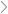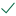Questions & AnswersMicroeconomicsRefer to the graph above to answer this question.  All of the...

QuestionAnswered step-by-step

# Refer to the graph above to answer this question.  All of the...

Refer to the graph above to answer this question. All of the following statements except one are correctWhich is the exception?

Select one:

A.Increasing returns to scale are experienced when output is increased from Q1 to Q4.

B.Plant 2 achieves minimum efficient scale.

C.Output level Q3 can be produced for the same cost in plant 1 and in plant 2.

D.Output level Q3 is economic capacity for plant size 1.

E.Plant 1 has excess capacity at output level Q1.

Clear my choice

Question 6

Points out of 1.00

Flag question

Question text

Refer to the graph above to answer this question. All of the following statements except one are correctWhich is the exception?

Select one:

A.The long-run average cost curve illustrates both increasing and constant returns to scale.

B.Constant returns to scale exist between outputs Q4 and Q5.

C.AC1, AC2 and AC3 are long-run average cost curves.

D.Both the short-run and the long run-costs are illustrated in this graph.

E.An increase in output from Q1 to Q3 could occur in plant 1.

Clear my choice

Question 7

Points out of 1.00

Flag question

Question text

Refer to the graph above to answer this question. Between what output levels do increasing returns to scale exist?

Select one:

A.Q1 and Q5.

B.Q1 and Q3 only.

C.Q1 and Q2 only.

D.Q1 and Q4.

E.Q4 and Q5.

Clear my choice

Question 8

Points out of 1.00

Flag question

Question text

Refer to the graph above to answer this question. Between what output levels do constant returns to scale exist?

Select one:

A.Q1 and Q4.

B.Q1 and Q3.

C.Q4 and Q5.

D.Q1 and Q5.

E.Q1 and Q2.

Refer to the graph above to answer this question. Can/should this firm produce an output of 200 at an average total cost of \$40?

Select one:

A.No, this combination would be unobtainable because production is inefficient.

B.No, this combination is unobtainable given present resource prices and the current state of technology.

C.Yes, and that output would be economic capacity.

D.Yes, but production would be inefficient.

Clear my choice

Question 10

Points out of 1.00

Flag question

Question text

Refer to the graph above to answer this question. Can/shouldt his firm produce an output of 200 at an average total cost of \$30?

Select one:

A.Yes, and that output would be economic capacity.

B.Yes, but production would be inefficient.

C.Yes, and the firm is experiencing economies of scale.

D.No, this combination is unobtainable because production would be inefficient.

E.No, this combination is unobtainable given present resource prices and the current state of technology.

Clear my choice

Question 11

Points out of 1.00

Flag question

Question text

Refer to the graph above to answer this question. Can/shouldthis firm produce an output of 200 at an average total cost of \$20?

** hint - READ carefully and think about the LRAC concept and how it is created!

Select one:

A.Yes, and that output is economic capacity.

B.Yes, but production would be inefficient.

C.No, this combination is unobtainable because production would be inefficient.

D.No, this combination would be unobtainable given present resource prices and the current state of technology.Solved by verified expert
<p>sectetur adipiscing elit. Nam lacini</p>sectetur adipiscing elit. Nam lacinia pulvinar tortor nec facilisis. Pellentesque dapibus efficitur laoreet. Nam risus ante, dapibus a molestie consequat, ultrices ac magna. Fusce dui lectus, congue vel laoreet ac, dictum vitae odio. Donec aliquet. Lorem ipsum dolor sit amet, consectetu10,000 step-by-step explanations